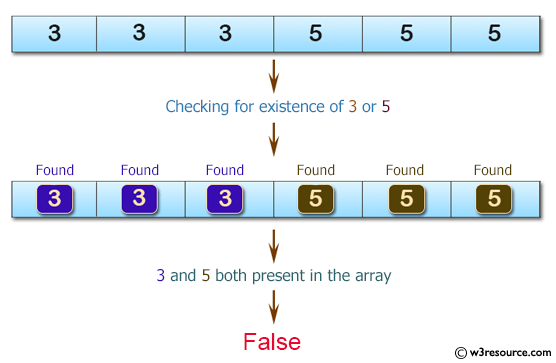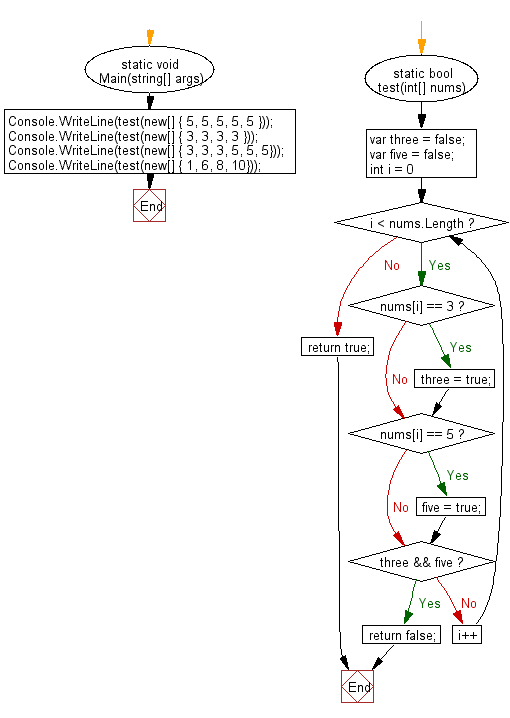﻿ C# - Check whether a given of integers contains no 3 or a 5# C# Sharp Basic Algorithm Exercises: Check whether a given array of integers contains no 3 or a 5

## C# Sharp Basic Algorithm: Exercise-118 with Solution

Write a C# Sharp program to check whether a given array of integers contains no 3 or a 5.

Pictorial Presentation:Sample Solution:

C# Sharp Code:

``````using System;
namespace exercises
{
class Program
{
static void Main(string[] args)
{
Console.WriteLine(test(new[] { 5, 5, 5, 5, 5 }));
Console.WriteLine(test(new[] { 3, 3, 3, 3 }));
Console.WriteLine(test(new[] { 3, 3, 3, 5, 5, 5}));
Console.WriteLine(test(new[] { 1, 6, 8, 10}));
}
static bool test(int[] nums)
{
var three = false;
var five = false;

for (int i = 0; i < nums.Length; i++)
{
if (nums[i] == 3) {three = true;}
if (nums[i] == 5) {five  = true;}
if (three && five) return false;
}
return true;
}
}
}
```
```

Sample Output:

```True
True
False
True```

Flowchart:C# Sharp Code Editor:

Improve this sample solution and post your code through Disqus

What is the difficulty level of this exercise?

Test your Programming skills with w3resource's quiz.

﻿